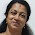## Thursday, August 25, 2011

### CBSE Class X Self Evaluation Tests For Maths : Real Numbers

1. The values of the remainder r, when a positive integer a is divided by 3 are 0 and 1 only. Justify your answer

No. According to Euclid’s division lemma,
a = 3q + r, where 0 r < 3 and r is an integer. Therefore, the values of r can be 0, 1 or 2.

2. Can the number 6n, n being a natural number, end with the digit 5? Give reasons.

No, because 6n = (2 × 3)n = 2n × 3n, so the only primes in the factorization of 6n are 2 and 3, and not 5. Hence, it cannot end with the digit 5.

3. Write whether every positive integer can be of the form 4q + 2, where q is an integer. Justify your answer.

No, because an integer can be written in the form 4q, 4q+1, 4q+2, 4q+3.

4. “The product of two consecutive positive integers is divisible by 2”. Is this statement true or false? Give reasons.

True, because n (n+1) will always be even, as one out of n or (n+1) must be even

5. “The product of three consecutive positive integers is divisible by 6”. Is this statement true or false”? Justify your answer.

True, because n (n+1) (n+2) will always be divisible by 6, as at least one of the factors will be divisible by 2 and at least one of the factors will be divisible by 3.

6. Write whether the square of any positive integer can be of the form 3m + 2, where m is a natural number. Justify your answer.

No. Since any positive integer can be written as 3q, 3q+1, 3q+2,
therefore, square will be 9q2 = 3m, 9q2 + 6q + 1 = 3 (3q2 + 2q) + 1 = 3m + 1, 9q2 + 12q + 3 + 1 = 3m + 1.

7. A positive integer is of the form 3q + 1, q being a natural number. Can you write its square in any form other than 3m + 1, i.e., 3m or 3m + 2 for some integer m? Justify your answer.

No. (3q + 1)2 = 9q2 + 6q + 1 = 3 (3q2 + 2q) = 3m + 1.

8. The numbers 525 and 3000 are both divisible only by 3, 5, 15, 25 and 75. What is HCF (525, 3000)? Justify your answer.

HCF = 75, as HCF is the highest common factor

9. Explain why 3 × 5 × 7 + 7 is a composite number.

3×5×7+7 = 7 (3×5 + 1) = 7 (16), which has more than two factors

10. Can two numbers have 18 as their HCF and 380 as their LCM? Give reasons.

No, because HCF (18) does not divide LCM (380).

11. Without actually performing the long division, find if 987/10500 will have terminating or non-terminating (repeating) decimal expansion. Give reasons for your answer.

Terminating decimal expansion, because 987/ 10500 = 47/ 500  and 500 =53 22

12. A rational number in its decimal expansion is 327/7081. What can you say about the prime factors of q, when this number is expressed in the form p/q ? Give reasons.
Since 327.7081 is a terminating decimal number, so q must be of the form 2m.5n;m, n are natural numbers

1.2.